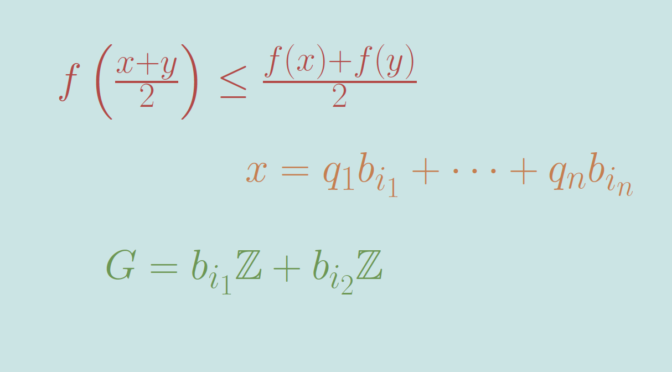# A discontinuous midpoint convex function

Let’s recall that a real function $$f: \mathbb R \to \mathbb R$$ is called convex if for all $$x, y \in \mathbb R$$ and $$\lambda \in [0,1]$$ we have $f((1- \lambda) x + \lambda y) \le (1- \lambda) f(x) + \lambda f(y)$ $$f$$ is called midpoint convex if for all $$x, y \in \mathbb R$$ $f \left(\frac{x+y}{2}\right) \le \frac{f(x)+f(y)}{2}$ One can prove that a continuous midpoint convex function is convex. Sierpinski proved the stronger theorem, that a real-valued Lebesgue measurable function that is midpoint convex will be convex.

Can one find a discontinuous midpoint convex function? The answer is positive but requires the Axiom of Choice. Why? Because Robert M. Solovay constructed a model of Zermelo-Fraenkel set theory (ZF), exclusive of the axiom of choice where all functions are Lebesgue measurable. Hence convex according to Sierpinski theorem. And one knows that convex functions defined on $$\mathbb R$$ are continuous.

Referring to my previous article on the existence of discontinuous additive map, let’s use a Hamel basis $$\mathcal B = (b_i)_{i \in I}$$ of $$\mathbb R$$ considered as a vector space on $$\mathbb Q$$. Take $$i_1 \in I$$, define $$f(i_1)=1$$ and $$f(i)=0$$ for $$i \in I\setminus \{i_1\}$$ and extend $$f$$ linearly on $$\mathbb R$$. $$f$$ is midpoint convex as it is linear. As the image of $$\mathbb R$$ under $$f$$ is $$\mathbb Q$$, $$f$$ is discontinuous as explained in the discontinuous additive map counterexample.

Moreover, $$f$$ is unbounded on all open real subsets. By linearity, it is sufficient to prove that $$f$$ is unbounded around $$0$$. Let’s consider $$i_1 \neq i_2 \in I$$. $$G= b_{i_1} \mathbb Z + b_{i_2} \mathbb Z$$ is a proper subgroup of the additive $$\mathbb R$$ group. Hence $$G$$ is either dense of discrete. It cannot be discrete as the set of vectors $$\{b_1,b_2\}$$ is linearly independent. Hence $$G$$ is dense in $$\mathbb R$$. Therefore, one can find a non vanishing sequence $$(x_n)_{n \in \mathbb N}=(q_n^1 b_{i_1} + q_n^2 b_{i_2})_{n \in \mathbb N}$$ (with $$(q_n^1,q_n^2) \in \mathbb Q^2$$ for all $$n \in \mathbb N$$) converging to $$0$$. As $$\{b_1,b_2\}$$ is linearly independent, this implies $$\vert q_n^1 \vert, \vert q_n^2 \vert \underset{n\to+\infty}{\longrightarrow} \infty$$ and therefore $\lim\limits_{n \to \infty} \vert f(x_n) \vert = \lim\limits_{n \to \infty} \vert f(q_n^1 b_{i_1} + q_n^2 b_{i_2}) \vert = \lim\limits_{n \to \infty} \vert q_n^1 \vert = \infty.$